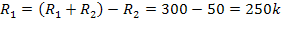# DC & AC Voltage Measurement

USB-IO adapter sends the results of the measurement to PC software. The voltage can be measured on demand (when PC software requests to measure voltage on the specified analog input pin) or periodically (each in milliseconds).

You can use either the USB-IO adapter positive and negative supply voltage (VDD and VSS) or the voltage level on the C.6 (VRefHi) and C.5 (VRefLow) pins as analog reference voltage.

## DC Voltage Measurement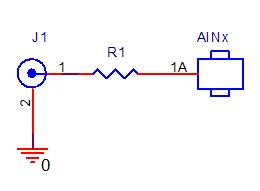If you want to measure voltage in wider range, add an external resistive divider: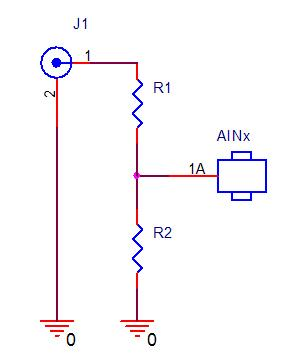The resistors used in this divider should meet the following requirements:

The total input resistance (R1+R2) should be at least 10 times greater than the output impedance of the connected circuit;

The total input resistance (R1 + R2) should be at least 10 times less than the input impedance of the ADC;

The ratio between R1 and R2 should comply with the equation: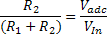where: Vadc is the maximum input voltage of the ADC (5V in our example); Vin is the maximum input voltage of the resistive divider (maximum output voltage of the connected circuit).

### Example:

You need to measure the voltage from 0V up to 30V. The output impedance of the connected circuit is 1k. The total resistance of R1 and R2 should be within:In current example we will set R1+R2 to 300k.

You can calculate the R1 and R2 values with the equation:## AC Voltage Measurement

You can measure the AC voltage by measuring the amplitude of the positive half-wave.

The possible connection to USB-IO adapter is illustrated by the following schematics: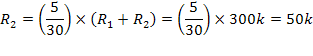In case of sinusoidal signal, you can get the actual voltage level with the following expression: